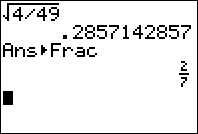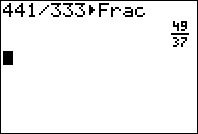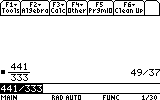SAT Prep #4: Using Your Calculator’s Fraction Features

Filed under Difficulty: Easy, SAT, Test Prep, TI-83 Plus, TI-84 Plus, TI-89.

Both the TI-83/TI-84 Plus and the TI-89 have built-in features for dealing with fractions, which can help to solve many of the fractions problems on the SAT. For example, on the TI-84, you can convert a decimal into a fraction by using the ▶Frac function. Simply type in a number, or else use 2nd to paste the Ans token and select your previous answer, then press to select the ▶Frac function, and again to evaluate.On the TI-89, almost all math is done symbolically, so if you evaluate an equation which does not involve decimals, you will receive an exact answer. If you want to convert a fraction into a decimal, enter the fraction, press , then to evaluate. Try the following problem:

Sample Problem: Student Produced Response. When the fraction 441/333 is reduced to lowest terms, what is the sum of the numerator and denominator?

This problem can take a fair amount of effort to work out by hand unless you immediately see how the numbers factor, and it’s easy to make a mistake. However, using your calculator makes it quick and painless. On the TI-84, type 441/333 then press to evaluate it as a fraction in lowest terms. On the TI-89, just type the fraction in and press , and it’s automatically simplified for you. 441/333 = 49/37, so the answer is 49+37 = 86.One more tip: if you would like to convert a decimal to a fraction on the TI-84, you can use the exact() function, which converts a decimal into its “exact” fractional form. This function can be reached by pressing 2nd to select the math menu, then pressing . To learn more about the exact function and other techniques for dealing with fractions on your calculator and for more practice, check out our book!